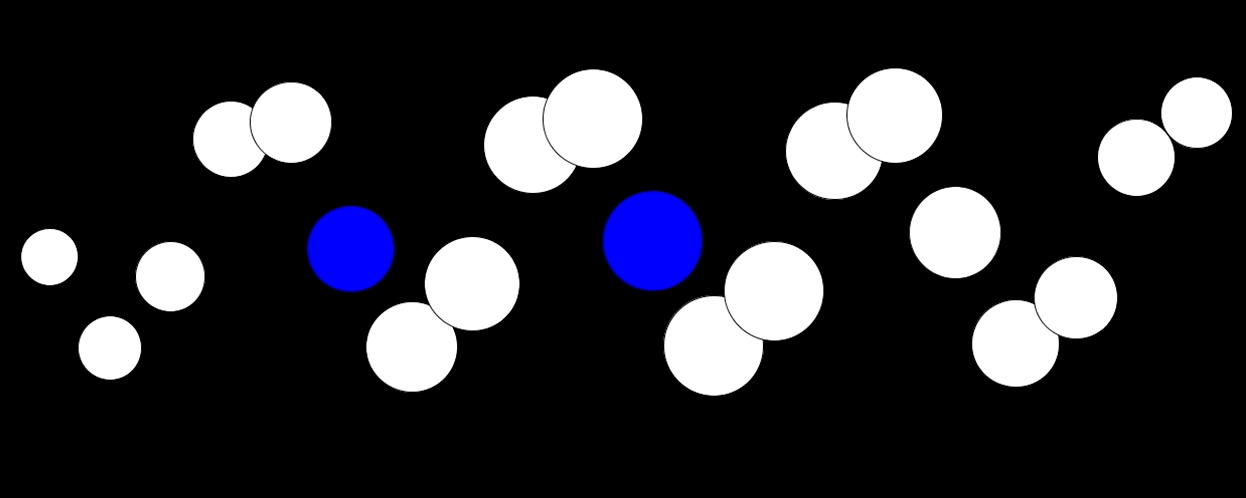# Latest Posts# Processing

I’ve recently been playing with Processing. Here are the results. I did experiment with triggering some MIDI notes at the center threshold but it didn’t sound as good as I wanted. I’ll expand on that once I get a bit more time.

## The Source

```import themidibus.*; //Import the library
MidiBus myBus; // The MidiBus
float b = 20;
float d = 0;

void setup () {
size(1280, 720);
frameRate(30);
MidiBus.list();
myBus = new MidiBus(this, "Bus 1", "Bus 1");
}

void draw() {

int icCount = 20;
double number = Math.PI * 2 / icCount;
background(0);

for (int n=1; n<= icCount; n++) { double x = 300 + ((Math.sin(number * (17-(n/ Math.PI)) * b)) * 120); double y = (n * 60) + 20; double s = 50 * (Math.sin(b*n) +1); //if(n % 4 ==0) { double check = Math.sin(number * (17-(n/ Math.PI)) * b); if (check >=0.1 && check <=0.2) {
//myBus.sendNoteOn(1, n + 60, 12);
fill(#0000ff);
} else {
//myBus.sendNoteOff(1, n + 60, 12);
fill(#ffffff);
}
//}

ellipse((float)y,(float)x,(float)s,(float)s);
}

b += 0.02;
d += 0.04;

}
```

Check out the software here: https://processing.org/
Check out other sine programming examples here (JavaScript): https://roguelj.co.uk/toybox/

# HTML Canvas

Here is the JavaScript for the first of my experiments with the HTML Canvas tag.

``` var example; var context; var b =0;   function init() {   example = document.getElementById('canvas1'); context = example.getContext('2d'); b=0; draw();   }   function draw() {   context.fillStyle = "rgb(255,255,255)"; context.fillRect(0, 0, 400, 800);   var icCount = 12; var number = Math.PI * 2 / icCount;   for (n=1; n&lt;= icCount; n++) {   x = 200 + ((Math.sin(number * n * b)) * 150); y = 150 + ((Math.tan(number * n * b)) * 100);   context.fillStyle = 'rgb(0,0,0)';   context.beginPath(); context.arc(x, y, 25, 0, Math.PI*2, true); context.closePath(); context.fill();   }   b += 0.02;   setTimeout("draw()",33);   }```

Link to page:
https://roguelj.co.uk/sineart/a1.php

Link to other pages in this series:
https://roguelj.co.uk/toybox/

Link to the W3C School page about the HTML Canvas
https://www.w3schools.com/html/html5_canvas.asp

# Artstation

I’ve set up an Artstation portfolio, for my experiments with Blender and other associated bits of software. You can check it out at the following link https://roguelj.artstation.com

1 2 3 4 25